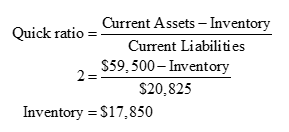# Crockett Electronics has a quick ratio of 2.00x, \$26,775 in cash, \$14,875 in accounts receivable, some inventory, total current assets of \$59,500, and total current liabilities of \$20,825. The company reported annual sales of \$400,000 in the most recent annual report.Over the past year, how often did Crockett Electronics sell and replace its inventory?

Question
96 views

Crockett Electronics has a quick ratio of 2.00x, \$26,775 in cash, \$14,875 in accounts receivable, some inventory, total current assets of \$59,500, and total current liabilities of \$20,825. The company reported annual sales of \$400,000 in the most recent annual report.

Over the past year, how often did Crockett Electronics sell and replace its inventory?

check_circle

Step 1

Quick ratio of the firm is 2.00x , current assets is \$59,500 and current liabilities is \$20825. Therefore, inventory of the firm can be calculated as below:Step 2

Inventory turnover helps in determining the number of times inventory is sold and replaced in each period. It is calculated as cost of goods sold divided by inventory; however, it can also give an approximate value when sales is divided by inve...

### Want to see the full answer?

See Solution

#### Want to see this answer and more?

Solutions are written by subject experts who are available 24/7. Questions are typically answered within 1 hour.*

See Solution
*Response times may vary by subject and question.
Tagged in